# Clarification and Simplfication on current flow in a DC Series/Parallel Circuit

#### Kuturan

Joined Jan 11, 2020
11
As title say, I am struggling with understanding this problem and how current is flowing through the circuit. I have the answers already, and while the values of Va and Vb (-6V and -20V) makes sense to me due to their direct connections to their respective sources, I do not understand how the current I and Voltage Vab was obtained.

I have tried mesh analysis with this as well as redrawing the circuit, with the former giving me incorrect results for I and Vab and me being stuck on the latter in regards to the placement of the 2Ohm resistor.

A question like this seems really easy for this forum so I apologize in advance if this is a dumb question, I am trying to review the fundamentals for DC circuits and their respective analysis methods/theorems before I move onto RL and AC circuits.

I appreciate any help and I can get and thank you in advance.

#### crutschow

Joined Mar 14, 2008
31,128
Did you try to use superposition to solve for the values?

#### WBahn

Joined Mar 31, 2012
27,900
As title say, I am struggling with understanding this problem and how current is flowing through the circuit. I have the answers already, and while the values of Va and Vb (-6V and -20V) makes sense to me due to their direct connections to their respective sources, I do not understand how the current I and Voltage Vab was obtained.

I have tried mesh analysis with this as well as redrawing the circuit, with the former giving me incorrect results for I and Vab and me being stuck on the latter in regards to the placement of the 2Ohm resistor.

A question like this seems really easy for this forum so I apologize in advance if this is a dumb question, I am trying to review the fundamentals for DC circuits and their respective analysis methods/theorems before I move onto RL and AC circuits.

I appreciate any help and I can get and thank you in advance.
Va and Vb are not -6 V and -20 V just because they are connected directly to sources of those voltages. The key thing is that the OTHER side of each of those sources is connected to the 0 V node. A voltage source establishes a voltage ACROSS the source. If ONE side of the source is at a known voltage, then the voltage at the other side of the source can be determined by adding the voltage across the source (the unknown side relative to the known side) to the voltage at the known side.

The dual-subscript convention say that Vxy is the voltage at node X relative to the voltage at node Y, so

Vxy = Vx - Vy

Turning to you redrawing attempt, you need to be sure that each component is still connected to the same node. A simple way of doing that is to number or color all of the nodes and then see if each component is still connected to the same nodes. Also, you need to carry across all of the information from the original circuit, including ground reference designators, node labels, and current/voltage designators. Another thing to be very careful about in this particular problem is that you are not asked for the current in a component, but rather the current in a particular second of wire of one node, so your redrawn circuit has to preserve that information (unless you just want to solve for other quantities and then come back to the original circuit to find that current, which is a perfectly valid and reasonable approach).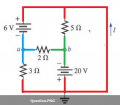No compare this to your redraw attempt.Do you see the problem?

#### Kuturan

Joined Jan 11, 2020
11
Va and Vb are not -6 V and -20 V just because they are connected directly to sources of those voltages. The key thing is that the OTHER side of each of those sources is connected to the 0 V node. A voltage source establishes a voltage ACROSS the source. If ONE side of the source is at a known voltage, then the voltage at the other side of the source can be determined by adding the voltage across the source (the unknown side relative to the known side) to the voltage at the known side.

The dual-subscript convention say that Vxy is the voltage at node X relative to the voltage at node Y, so

Vxy = Vx - Vy

Turning to you redrawing attempt, you need to be sure that each component is still connected to the same node. A simple way of doing that is to number or color all of the nodes and then see if each component is still connected to the same nodes. Also, you need to carry across all of the information from the original circuit, including ground reference designators, node labels, and current/voltage designators. Another thing to be very careful about in this particular problem is that you are not asked for the current in a component, but rather the current in a particular second of wire of one node, so your redrawn circuit has to preserve that information (unless you just want to solve for other quantities and then come back to the original circuit to find that current, which is a perfectly valid and reasonable approach).

View attachment 196754

No compare this to your redraw attempt.
View attachment 196755

Do you see the problem?
Thank you for the color diagram, it helped clarify a few things for another redrawing which I posted as of this reply. I am however, a bit confused by what you mean in regards to voltage connection for pts a and b towards the 0V node. Could you please clarify a little bit on what you mean by this?

#### Kuturan

Joined Jan 11, 2020
11
Did you try to use superposition to solve for the values?
For the Superposition Theorem, I could use it with a redrawn circuit, but I am unsure as to how I would find voltages of the individual ab nodes and the subsequent Vab voltage as the question doesn't ask for component values.

#### WBahn

Joined Mar 31, 2012
27,900
Thank you for the color diagram, it helped clarify a few things for another redrawing which I posted as of this reply. I am however, a bit confused by what you mean in regards to voltage connection for pts a and b towards the 0V node. Could you please clarify a little bit on what you mean by this?
Think of voltages like elevations and heights. When I ask for the height of an object, I'm asking for the distance from one side of the object, say the top, to the other side, say the bottom. When I ask for the elevation of an object, I'm asking for the distance between a particular side of the object and some common reference point, such as sea level.

So now let's say that I have a 50 m tall object and I set a 10 m tall object on top of it. What is the elevation of the bottom of the 10 m object? You don't know. You can't say that it's elevation is 50 m just because it is connected to a 50 m tall object. But IF you know that the OTHER end of the 50 m tall object is fixed at the 0 m reference point (sea level), THEN you can say that the elevation of the bottom of the 10 m object is 50 m.

Voltage work the same way -- and for the same reason. Elevation and height are measures of gravitational potential while voltage is a measure of electrical potential.

#### WBahn

Joined Mar 31, 2012
27,900
For the Superposition Theorem, I could use it with a redrawn circuit, but I am unsure as to how I would find voltages of the individual ab nodes and the subsequent Vab voltage as the question doesn't ask for component values.
The whole idea behind Superposition is that the voltage on a particular node, or the current through a particular branch, is simply the sum of that same quantity over all of the individual circuits (in which each independent source is not set to zero in exactly one of them).

#### Kuturan

Joined Jan 11, 2020
11
Think of voltages like elevations and heights. When I ask for the height of an object, I'm asking for the distance from one side of the object, say the top, to the other side, say the bottom. When I ask for the elevation of an object, I'm asking for the distance between a particular side of the object and some common reference point, such as sea level.

So now let's say that I have a 50 m tall object and I set a 10 m tall object on top of it. What is the elevation of the bottom of the 10 m object? You don't know. You can't say that it's elevation is 50 m just because it is connected to a 50 m tall object. But IF you know that the OTHER end of the 50 m tall object is fixed at the 0 m reference point (sea level), THEN you can say that the elevation of the bottom of the 10 m object is 50 m.

Voltage work the same way -- and for the same reason. Elevation and height are measures of gravitational potential while voltage is a measure of electrical potential.
I think I can understand what you mean, basically the voltage is the difference of two pts (that being the 50m tall object minus the 0m sea level) right? If that's the case, where are you getting the 0V from?

I'm going to try to explain what I think you mean in my own words below.

Is it just the case where if a point is not connected in series with another source in one direction, then the voltage from that direction to the point is automatically 0? Kinda confused here though, since components connected in parallel share voltage with the source they are connected in parallel with no? Perhaps I'm confusing voltage with current here?

However, putting that aside and assuming this is the case, we have a direct connection to a voltage source from another direction of the point, we get our final answer for voltage which is E1-E2 with E2 being 0 due to a lack of direct connection, we get our answer which is just E1.

I have attached a photo approximation of what I am thinking based on the circuit redrawing that I made thanks to your help and would appreciate your take on this.

Thank you so much for your patience and advice, and I apologize in advance if I'm coming off as a bit of an idiot in the subject.

#### WBahn

Joined Mar 31, 2012
27,900
I think I can understand what you mean, basically the voltage is the difference of two pts (that being the 50m tall object minus the 0m sea level) right? If that's the case, where are you getting the 0V from?
This is a central point that many people struggle with.

Just like we get to pick one elevation and declare that it is the zero elevation reference -- such as mean "sea level" or "ground level" or "above the table", we get to pick one point in the circuit and declare that it is the zero potential reference.

Voltages are ALWAYS a potential difference between two points (or along some path). When we say that a battery is 9V, what we really mean is that the voltage difference between the positive terminal and the negative terminal is 9V. Whenever we say that the voltage AT a particular point -- say Node 351 in some circuit -- is 31.5 V, what we are really saying is that the voltage at that point is 31.5 V higher than the voltage at the node that we declared was the zero potential reference point (what is commonly referred to as "ground", although "common" is a much more accurate term).

Digest that and then ask again if you still have some confusion.

#### Kuturan

Joined Jan 11, 2020
11
This is a central point that many people struggle with.

Just like we get to pick one elevation and declare that it is the zero elevation reference -- such as mean "sea level" or "ground level" or "above the table", we get to pick one point in the circuit and declare that it is the zero potential reference.

Voltages are ALWAYS a potential difference between two points (or along some path). When we say that a battery is 9V, what we really mean is that the voltage difference between the positive terminal and the negative terminal is 9V. Whenever we say that the voltage AT a particular point -- say Node 351 in some circuit -- is 31.5 V, what we are really saying is that the voltage at that point is 31.5 V higher than the voltage at the node that we declared was the zero potential reference point (what is commonly referred to as "ground", although "common" is a much more accurate term).

Digest that and then ask again if you still have some confusion.
I think I can understand somewhat. You're basically saying voltage is the voltage at that point minus the voltage at whatever reference point you've set to as ground in the circuit.

I kinda think I might be overthinking this. If I apply this concept to my problem for Va and Vb, we get -6V and -20V respectively from one side, where would I be getting the 0V from in this particular circuit?

From my redrawing, if you apply Kirchhoff's Voltage Law to each individual loop consisting of the voltage sources, a resistor, and pts a and b separately, I can understand where you get the voltage values (basically voltage drop from 6V to fufill the conditions of KVL which makes it -6V etc.) but there's more than one connection when you put it together so I'm still confused as to where the 0V is coming from.

As for using Superposition Theorem, I might be applying it wrong but I've applied it to the redrawn circuit I've made but I seem to be getting a value of 7A (10A-3A) versus the book's correct answer which is 9. Perhaps I redrawn something wrong?

#### WBahn

Joined Mar 31, 2012
27,900
I think I can understand somewhat. You're basically saying voltage is the voltage at that point minus the voltage at whatever reference point you've set to as ground in the circuit.

I kinda think I might be overthinking this. If I apply this concept to my problem for Va and Vb, we get -6V and -20V respectively from one side, where would I be getting the 0V from in this particular circuit?
You do NOT get -6 V "from one side". That has no meaning -- you appear to still be thinking that JUST because you are connected to the negative terminal of an X volt source that the voltage is -X volts. That's not true.

Consider the following: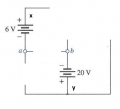All the 6 V source does is impose a constraint that

Vxa = Vx - Va = 6 V

Solving for Va we get

Va = Vx - 6 V

Similarly, all the 20 V source does is impost the constraint

Vy - Vb = 20 V

and therefore

Vb = Vy - 20 V

If we want to know what Va or Vb is, relative to our 0 V reference, then we need to know what Vx and Vy are relative to that reference (or use some other path to the reference).

If we want to know what Vab is, however, we don't necessarily need to know what either of them is, relative to 0 V, if we can determine what Vxy is (Vx relative to Vy).

Vab = Va - Vb = (Vx - 6 V) - (Vy - 20 V) = Vxy + 14 V

So let's look at a bit more of the diagram.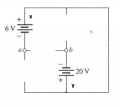Here we can see that node x is the same as node y, so there is no voltage difference between them (because at this level we assume wires are equipotential surfaces). So Vxy = 0

So we can find Vab even though we don't know what, relative to the 0 V reference, Va, Vb, Vx, or Vy is -- in no small part because this diagram doesn't define what point we are choosing to call 0 V.

So let's look at a bit more of the diagram.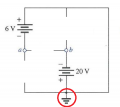The addition of that symbol (in this context) does one thing and one thing only -- it defines the voltage on the node it is attached to as being 0 V.

That means that Vx = Vy = 0V and NOW we can conclude that

Va = Vx - 6 V = 0 V - 6 V = -6 V
Vb = Vy - 20 V = 0 V - 20 V = -20 V

Hopefully you now see that Va is not -6 V just because it happens to be connected to the negative terminal of a 6 V battery, but because the OTHER side of that battery is at 0 V.

For instance, what if I did the following: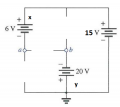What is Va now?

We still have

Vx - Va = 6 V (due to the 6 V battery)
Vy - Vb = 20 V (due to the 20 V battery)
Vy = 0 (due to the ground symbol)

But now we also have

Vx - Vy = 15 V (due to the 15 V battery)
Vx = Vy + 15 V

So

Va = Vx - 6 V
Va = Vx - 6 V
Va = Vy + 15 V - 6 V
Va = 0 V + 15 V - 6 V
Va = 9 V

•Kuturan

#### RBR1317

Joined Nov 13, 2010
706
While this may be a somewhat perverse circuit, once the voltage at each node is established you will then have the voltage drop across each resistor, and from that the current flowing in each branch. But realize that a theoretical voltage source will normally source current, but can also sink current (in the reverse direction) to maintain the specified voltage in the resistor network.

#### Kuturan

Joined Jan 11, 2020
11
You do NOT get -6 V "from one side". That has no meaning -- you appear to still be thinking that JUST because you are connected to the negative terminal of an X volt source that the voltage is -X volts. That's not true.

Consider the following:

View attachment 196951

All the 6 V source does is impose a constraint that

Vxa = Vx - Va = 6 V

Solving for Va we get

Va = Vx - 6 V

Similarly, all the 20 V source does is impost the constraint

Vy - Vb = 20 V

and therefore

Vb = Vy - 20 V

If we want to know what Va or Vb is, relative to our 0 V reference, then we need to know what Vx and Vy are relative to that reference (or use some other path to the reference).

If we want to know what Vab is, however, we don't necessarily need to know what either of them is, relative to 0 V, if we can determine what Vxy is (Vx relative to Vy).

Vab = Va - Vb = (Vx - 6 V) - (Vy - 20 V) = Vxy + 14 V

So let's look at a bit more of the diagram.

View attachment 196953
Here we can see that node x is the same as node y, so there is no voltage difference between them (because at this level we assume wires are equipotential surfaces). So Vxy = 0

So we can find Vab even though we don't know what, relative to the 0 V reference, Va, Vb, Vx, or Vy is -- in no small part because this diagram doesn't define what point we are choosing to call 0 V.

So let's look at a bit more of the diagram.
View attachment 196954
The addition of that symbol (in this context) does one thing and one thing only -- it defines the voltage on the node it is attached to as being 0 V.

That means that Vx = Vy = 0V and NOW we can conclude that

Va = Vx - 6 V = 0 V - 6 V = -6 V
Vb = Vy - 20 V = 0 V - 20 V = -20 V

Hopefully you now see that Va is not -6 V just because it happens to be connected to the negative terminal of a 6 V battery, but because the OTHER side of that battery is at 0 V.

For instance, what if I did the following:

View attachment 196959

What is Va now?

We still have

Vx - Va = 6 V (due to the 6 V battery)
Vy - Vb = 20 V (due to the 20 V battery)
Vy = 0 (due to the ground symbol)

But now we also have

Vx - Vy = 15 V (due to the 15 V battery)
Vx = Vy + 15 V

So

Va = Vx - 6 V
Va = Vx - 6 V
Va = Vy + 15 V - 6 V
Va = 0 V + 15 V - 6 V
Va = 9 V
Thanks man, I'm sorry for making you explain it to this level of detail. So the reference node in this case just happens to be zero because of the ground in the diagram right?

#### Kuturan

Joined Jan 11, 2020
11
You do NOT get -6 V "from one side". That has no meaning -- you appear to still be thinking that JUST because you are connected to the negative terminal of an X volt source that the voltage is -X volts. That's not true.

Consider the following:

View attachment 196951

All the 6 V source does is impose a constraint that

Vxa = Vx - Va = 6 V

Solving for Va we get

Va = Vx - 6 V

Similarly, all the 20 V source does is impost the constraint

Vy - Vb = 20 V

and therefore

Vb = Vy - 20 V

If we want to know what Va or Vb is, relative to our 0 V reference, then we need to know what Vx and Vy are relative to that reference (or use some other path to the reference).

If we want to know what Vab is, however, we don't necessarily need to know what either of them is, relative to 0 V, if we can determine what Vxy is (Vx relative to Vy).

Vab = Va - Vb = (Vx - 6 V) - (Vy - 20 V) = Vxy + 14 V

So let's look at a bit more of the diagram.

View attachment 196953
Here we can see that node x is the same as node y, so there is no voltage difference between them (because at this level we assume wires are equipotential surfaces). So Vxy = 0

So we can find Vab even though we don't know what, relative to the 0 V reference, Va, Vb, Vx, or Vy is -- in no small part because this diagram doesn't define what point we are choosing to call 0 V.

So let's look at a bit more of the diagram.
View attachment 196954
The addition of that symbol (in this context) does one thing and one thing only -- it defines the voltage on the node it is attached to as being 0 V.

That means that Vx = Vy = 0V and NOW we can conclude that

Va = Vx - 6 V = 0 V - 6 V = -6 V
Vb = Vy - 20 V = 0 V - 20 V = -20 V

Hopefully you now see that Va is not -6 V just because it happens to be connected to the negative terminal of a 6 V battery, but because the OTHER side of that battery is at 0 V.

For instance, what if I did the following:

View attachment 196959

What is Va now?

We still have

Vx - Va = 6 V (due to the 6 V battery)
Vy - Vb = 20 V (due to the 20 V battery)
Vy = 0 (due to the ground symbol)

But now we also have

Vx - Vy = 15 V (due to the 15 V battery)
Vx = Vy + 15 V

So

Va = Vx - 6 V
Va = Vx - 6 V
Va = Vy + 15 V - 6 V
Va = 0 V + 15 V - 6 V
Va = 9 V
Oh and also, I have another question about Node 2. Would current be different at Node 2 compared to Node 1 as Node 2 seems to be connected to the 20V source in parallel? I'm assuming voltage is the same because of shared voltage between the nodes.

#### WBahn

Joined Mar 31, 2012
27,900
Thanks man, I'm sorry for making you explain it to this level of detail. So the reference node in this case just happens to be zero because of the ground in the diagram right?
The reference node is always zero -- that's what makes it the reference node.

But in this case the reference node just happens to be the node that is connected to the positive terminal of the sources connected to nodes 'a' and 'b'.

#### WBahn

Joined Mar 31, 2012
27,900
Oh and also, I have another question about Node 2. Would current be different at Node 2 compared to Node 1 as Node 2 seems to be connected to the 20V source in parallel? I'm assuming voltage is the same because of shared voltage between the nodes.
Which node is Node 2? Which node is Node 1?

Don't refer to quantities that you haven't defined -- people have no way of knowing what you are talking about.

#### RBR1317

Joined Nov 13, 2010
706